# 3.10 Shapes of Molecules - VSEPR Theory and Valence Bond Theory

## VSEPR Theory

Valence Shell Electron Pair Repulsion Theory uses the basic idea that electron pairs are mutually repulsed to predict the arrangement of electron pairs around a central atom (an atom that has at least two other atoms bonded directly to it). The key to correctly applying VSEPR Theory is to start with a correct Lewis dot structure. From a correct Lewis dot structure, it is a straightforward process to determine the shape of a molecule or polyatomic ion by determining the arrangement of electron pairs around every central atom in the molecule or polyatomic ion.

The step-by-step process to determine the shape around each central atom is

1. Draw the Lewis dot structure.

2. Apply the following analysis to each separate, central atom (where a central atom is an atom with two or more other atoms bonded directly to it.)

a) Find the number of "things attached" to the central atom, where a "thing attached" is either an atom or a non-bonding electron pair.

(Actually, you are counting the number of hybrid orbitals on the central atom, but that is the next theory to be discussed.)

It is important to remember that you are not counting bonds!

b) Find the Electron Group Arrangement (EGA) in the table below

# of Things Attached Electron Group Arrangement Ideal Bond Angle Hybridization of Central Atom
2 line (linear shape) 180 sp
3 trigonal plane( trigonal planar shape) 120 sp2
4 tetrahedron (tetrahedral shape) 109.5 sp3

c) Find the Molecular Geometry (MG) of the particle, determined by counting up the number of atoms and the number of lone pairs and using this table:

Hybridization of Central Atom

(see Valence Bond Theory)

# of atoms attached # of lone pairs attached Molecular Geometry Bond angle Example
sp hybridized central atom:
2 0 line 180 CO2
sp2 hybridized central atom:
3 0 trigonal plane 120 BF3
2 1 bent <120 SO2
sp3 hybridized central atom:
4 0 tetrahedron 109.5 CH4
3 1 trigonal pyramid <109.5 NH3
2 2 bent <109.5 H2O

Example $$\PageIndex{1}$$

Determine the Electron Group Arrangement and Molecular Geometry about the central atom(s) in a) OF2 and b) CH3CN.

Solution

a) The Lewis dot structure of OF2 is(leaving off the lone pairs on the non-central F atoms.)

There are 2 atoms and 2 lone pairs attached to the central O atom, for a total of 4 "things attached."

From the two tables above, we can see that the O atom must have an EGA of a tetrahedron, and its MG is bent.

b) The Lewis dot structure of CH3CN isThere are 4 atoms and 0 lone pairs attached to the central C atom on the left, for a total of 4 "things attached."

From the two tables above, we can see that this C atom must have an EGA of a tetrahedron, and its MG is also a tetrahedron.

There are 2 atoms and 0 lone pairs attached to the central C atom on the right, for a total of 2 "things attached."

From the two tables above, we can see that this C atom must have an EGA of a line, and its MG is also a line.

Exercise $$\PageIndex{1}$$

Determine the Electron Group Arrangement and Molecular Geometry about the central atom(s) in a) PF3 and b) CH2NH.

a) The Lewis dot structure of PF3 is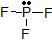(leaving off the lone pairs on the non-central F atoms.)

There are 3 atoms and 1 lone pair attached to the central P atom, for a total of 4 "things attached."

From the two tables above, we can see that the P atom must have an EGA of a tetrahedron, and its MG is a trigonal pyramid.

b)The Lewis dot structure of CH2NH is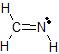There are 3 atoms and 0 lone pairs attached to the central C atom on the left, for a total of 3 "things attached."

From the two tables above, we can see that this C atom must have an EGA of a trigonal plane, and its MG is also a trigonal plane.

There are 2 atoms and 1 lone pair attached to the central N atom on the right, for a total of 3 "things attached."

From the two tables above, we can see that this N atom must have an EGA of a trigonal plane, and its MG is bent.

## Valence Bond Theory

The localized valence bond theory uses a process called hybridization, in which atomic orbitals are combined mathematically to produce sets of equivalent orbitals that are properly oriented to form bonds to create the common electron group arrangements. These new orbital combinations are called hybrid atomic orbitals because they are produced by combining (hybridizing) two or more atomic orbitals from the same atom.

#### Hybridizing One s Orbital and Three p Orbitals

Carbon atoms typically form four covalent bonds, and have electron group arrangement of a tetrahedron because there are four "things attached" to the central C atom. We can explain the tetrahedral shape by proposing that the 2s orbital and the three 2p orbitals on a carbon atom mix together to give a set of four degenerate sp3 (“s-p-three”) hybrid orbitals, each containing a single electron.

The large lobes of the hybridized orbitals are oriented toward the vertices of a tetrahedron, with 109.5° angles between them (Figure $$\PageIndex{1}$$). Like all the hybridized orbitals discussed earlier, the sp3 hybrid atomic orbitals are predicted to be equal in energy. Thus, methane (CH4) is a tetrahedral molecule with four equivalent C-H bonds.Figure $$\PageIndex{1}$$: Formation of sp3 Hybrid Orbitals. Combining one ns and three np atomic orbitals results in four sp3 hybrid orbitals oriented at 109.5° to one another in a tetrahedral arrangement.

An sp3 hybrid orbital looks a bit like half of a p orbital, and the four sp3 hybrid orbitals arrange themselves in space so that they are as far apart as possible. You can picture the nucleus as being at the centre of a tetrahedron (a triangularly based pyramid) with the orbitals pointing to the corners. For clarity, the nucleus is drawn far larger than it really is.What happens when the bonds are formed?

Remember that hydrogen's electron is in a 1s orbital - a spherically symmetric region of space surrounding the nucleus where there is some fixed chance (say 95%) of finding the electron. When a covalent bond is formed, the atomic orbitals (the orbitals in the individual atoms) merge to produce a new molecular orbital which contains the electron pair which creates the bond.Four molecular orbitals are formed, looking rather like the original sp3 hybrids, but with a hydrogen nucleus embedded in each lobe.

Hybrid orbital theory can be applied to molecules of ammonia (NH3) , which has three atoms and one lone pair attached to the central N atom. This means that the N atom is sp3 hybridized. Three sp3 orbitals form bonds with three H atoms, while the fourth orbital accommodates the lone pair of electrons. Similarly, H2O has an sp3 hybridized oxygen atom that uses two sp3 orbitals to bond to two H atoms, and two orbitals to accommodate the two lone pairs predicted by the VSEPR model. Such descriptions explain the approximately tetrahedral distribution of electron pairs on the central atom in NH3 and H2O.

#### Hybridizing One s Orbital and Two p Orbitals

Ethene, C2H4, is a molecule in which all of the atoms lie in a plane, with 120o bond angles between all of the atoms: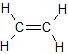To explain this structure, we can generate three equivalent hybrid orbitals on each C atom by combining the 2s orbital of the carbon and two of the three degenerate 2p orbitals. This mixing results in each C atom possessing a set of three equivalent hybrid orbitals with one electron each. The hybrid orbitals are degenerate and are oriented at 120° angles to each other (Figure $$\PageIndex{2}$$). Because the hybrid atomic orbitals are formed from one s and two p orbitals, carbon is said to be sp2 hybridized (pronounced “s-p-two” or “s-p-squared”).Figure $$\PageIndex{2}$$: Formation of sp2 Hybrid Orbitals. Combining one ns and two np atomic orbitals gives three equivalent sp2 hybrid orbitals in a trigonal planar arrangement; that is, oriented at 120° to one another.

Two of the sp2 hybrid atomic orbitals on each C atom can can overlap with the s orbitals on two H atoms. The third hybrid orbital on each C atom can overlap to form a sigma bond between the two C atoms. Both C atoms have one 2p orbital that has yet to be used for bonding. When the C atoms form a sigma bond and are pulled close together, a pi bond can also form between the two C atoms because the p-orbitals can overlap above and below the internuclear axis. The result to form a trigonal planar structure.

The entire bonding process looks like this (with the sp2 hybrid orbitals in bright green and the unhybridized p orbitals in red):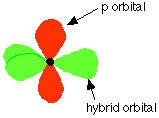The two carbon atoms and four hydrogen atoms would look like this before they joined together:The various atomic orbitals which are pointing towards each other now merge to give molecular orbitals, each containing a bonding pair of electrons. These are sigma bonds - just like those formed by end-to-end overlap of atomic orbitals in, say, ethane.The p orbitals on each carbon are not pointing towards each other, and so we'll leave those for a moment. In the diagram, the black dots represent the nuclei of the atoms. Notice that the p orbitals are so close that they are overlapping sideways.This sideways overlap also creates a $$\pi$$ bond.

For clarity, the sigma bonds are shown using lines - each line representing one pair of shared electrons. The various sorts of line show the directions the bonds point in. An ordinary line represents a bond in the plane of the screen (or the paper if you've printed it), a broken line is a bond going back away from you, and a wedge shows a bond coming out towards you.

Be clear about what a $$\pi$$ bond is. It is a region of space in which you can find the two electrons which make up the bond. Those two electrons can live anywhere within that space. It would be quite misleading to think of one living in the top and the other in the bottom.

#### Hybridizing One s Orbital and One p Orbital

Ethyne, C2H2, is a molecule in which all of the atoms lie in a straight line: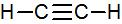To explain this structure, we can generate two equivalent hybrid orbitals on each C atom by combining the 2s orbital of carbon and any one of the three degenerate 2p orbitals.

By taking the sum and the difference of C 2s and 2pz atomic orbitals, for example, we produce two new orbitals with major and minor lobes oriented along the z-axes, as shown in Figure $$\PageIndex{3}$$.Figure $$\PageIndex{3}$$: The Formation of sp Hybrid Orbitals. Taking the sum and difference of an ns and an np atomic orbital where n = 2 gives two equivalent sp hybrid orbitals oriented at 180° to each other.

The nucleus resides just inside the minor lobe of each orbital. In this case, the new orbitals are called sp hybrids because they are formed from one s and one p orbital.

One sp hybrid orbital on each C atom can now form a sigma bond with one of the H atoms. The other sp hybrid orbital on each C atom can overlap to form a sigma bond between the two C atoms. Both C atoms have two 2p orbitals that have yet to be used for bonding. These orbitals are perpendicular to each other on each C atom, but when the C atoms form a sigma bond and are pulled close together, two pi bonds can also form between the two C atoms because one set of p-orbitals can overlap above and below the internuclear axis, and the other set of p orbitals can overlap in front of and behind the internuclear axis.

The entire bonding process looks like this (with the sp hybrid orbitals in bright green and the unhybridized p orbitals in red):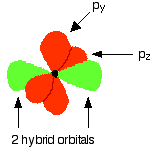Notice that the two green lobes are two different hybrid orbitals - arranged as far apart from each other as possible. Do not confuse them with the shape of a p orbital. The two carbon atoms and two hydrogen atoms would look like this before they joined together: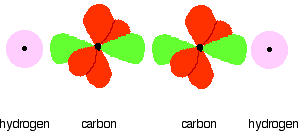The various atomic orbitals which are pointing towards each other now merge to give molecular orbitals, each containing a bonding pair of electrons. These are sigma bonds - just like those formed by end-to-end overlap of atomic orbitals in, say, ethane. The sigma bonds are shown as orange in the next diagram. The various p orbitals (now shown in slightly different reds to avoid confusion) are now close enough together that they overlap sideways.Sideways overlap between the two sets of p orbitals produces two pi bonds - each similar to the pi bond found in, say, ethene. These pi bonds are at 90° to each other - one above and below the molecule, and the other in front of and behind the molecule. Notice the different shades of red for the two different pi bonds.Example $$\PageIndex{1}$$

Use the VSEPR model to predict the number of electron pairs and molecular geometry in each compound and then describe the hybridization and bonding of all atoms except hydrogen.

1. H2S
2. CHCl3

Given: two chemical compounds

Asked for: number of electron pairs and molecular geometry, hybridization, and bonding

Strategy:

1. Using the VSEPR approach to determine the number of electron pairs and the molecular geometry of the molecule.
2. From the valence electron configuration of the central atom, predict the number and type of hybrid orbitals that can be produced. Fill these hybrid orbitals with the total number of valence electrons around the central atom and describe the hybridization.

Solution:

1. A H2S has four electron pairs around the sulfur atom with two bonded atoms, so the VSEPR model predicts a molecular geometry that is bent, or V shaped. B Sulfur hybridizes its 3s and 3p orbitals, to produce four sp3 hybrids. If the six valence electrons are placed in these orbitals, two have electron pairs and two are singly occupied. The two sp3 hybrid orbitals that are singly occupied are used to form S–H bonds, whereas the other two have lone pairs of electrons. Together, the four sp3 hybrid orbitals produce an approximately tetrahedral arrangement of electron pairs, which agrees with the molecular geometry predicted by the VSEPR model.
2. A The CHCl3 molecule has four valence electrons around the central atom. In the VSEPR model, the carbon atom has four electron pairs, and the molecular geometry is tetrahedral. B Carbon hybrides its 2s and 2p orbitals, to form four sp3 hybridized orbitals that are equal in energy. Eight electrons around the central atom (four from C, one from H, and one from each of the three Cl atoms) fill three sp3 hybrid orbitals to form C–Cl bonds, and one forms a C–H bond. Similarly, the Cl atoms, with seven electrons each in their 3s and 3p valence subshells, can be viewed as sp3 hybridized. Each Cl atom uses a singly occupied sp3 hybrid orbital to form a C–Cl bond and three hybrid orbitals to accommodate lone pairs.

Exercise $$\PageIndex{1}$$

Use the VSEPR model to predict the number of electron pairs and molecular geometry in each compound and then describe the hybridization and bonding of all atoms except hydrogen.

1. the BF4 ion
2. hydrazine (H2N–NH2)

B is sp3 hybridized; F is also sp3 hybridized so it can accommodate one B–F bond and three lone pairs. The molecular geometry is tetrahedral.

Each N atom is sp3 hybridized and uses one sp3 hybrid orbital to form the N–N bond, two to form N–H bonds, and one to accommodate a lone pair. The molecular geometry about each N is trigonal pyramidal.

The number of hybrid orbitals used by the central atom is the same as the number of electron pairs around the central atom, and thu sthe same as the number of "things attached" to the central atom.

### Hybridization Using d Orbitals

Hybridization is not restricted to the ns and np atomic orbitals, but we will not discuss the formation of the hybrid orbitals involving d orbitals.

### Summary

Hybridization increases the overlap of bonding orbitals and explains the molecular geometries of many species whose geometry cannot be explained using a VSEPR approach. The localized bonding model (called valence bond theory) assumes that covalent bonds are formed when atomic orbitals overlap and that the strength of a covalent bond is proportional to the amount of overlap. It also assumes that atoms use combinations of atomic orbitals (hybrids) to maximize the overlap with adjacent atoms. The combination of one s and one p orbital gives rise to two equivalent sp hybrids oriented at 180°, whereas the combination of one s and two or three p orbitals produces three equivalent sp2 hybrids or four equivalent sp3 hybrids, respectively. The spatial orientation of the hybrid atomic orbitals is consistent with the geometries predicted using the VSEPR model.

### Contributors

Jim Clark (Chemguide.co.uk)

Modified by Tom Neils (Grand Rapids Community College)